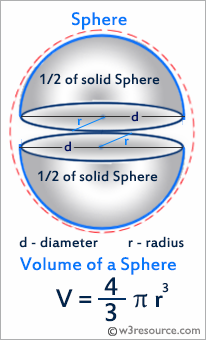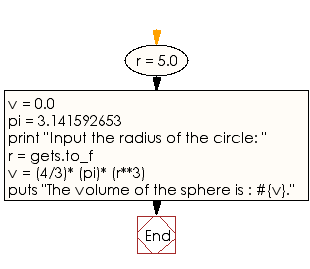﻿ Ruby Basic exercises: Compute the volume - w3resource# Ruby Basic Exercises: Compute the volume

## Ruby Basic: Exercise-14 with Solution

Write a Ruby program which accept the radius of the sphere as input and compute the volume.Ruby Code:

``````r = 5.0
v = 0.0
pi = 3.141592653
print "Input the radius of the circle: "
r = gets.to_f
v = (4/3)* (pi)* (r**3)
puts "The volume of the sphere is : #{v}."
``````

Output:

```Input the radius of the circle: The volume of the sphere is : 392.699081625.
```

Flowchart:Ruby Code Editor: